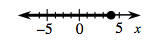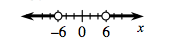### Home > CCA2 > Chapter 4 > Lesson 4.2.2 > Problem4-84

4-84.

Solve each of the following inequalities. Express the solutions algebraically and on a number line.

1. $3x-5\le7$

Solve the equation $3x-5\le7$ for $x$. Plot the boundary point on a number line. Test a point on each side of the boundary point. Shade the region that makes the inequality true.

$x\le4$1. $x^2+6>42$

See part (a). This inequality is quadratic, so look for two boundary points.

$x<−6$ or $x>6$